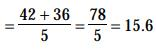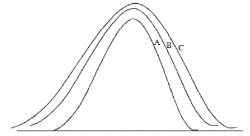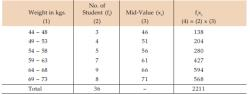Courses

# Test: Measures Of Central Tendency And Dispersion- 3

## 40 Questions MCQ Test Business Mathematics and Logical Reasoning & Statistics | Test: Measures Of Central Tendency And Dispersion- 3

Description
This mock test of Test: Measures Of Central Tendency And Dispersion- 3 for CA Foundation helps you for every CA Foundation entrance exam. This contains 40 Multiple Choice Questions for CA Foundation Test: Measures Of Central Tendency And Dispersion- 3 (mcq) to study with solutions a complete question bank. The solved questions answers in this Test: Measures Of Central Tendency And Dispersion- 3 quiz give you a good mix of easy questions and tough questions. CA Foundation students definitely take this Test: Measures Of Central Tendency And Dispersion- 3 exercise for a better result in the exam. You can find other Test: Measures Of Central Tendency And Dispersion- 3 extra questions, long questions & short questions for CA Foundation on EduRev as well by searching above.
QUESTION: 1

Solution:
QUESTION: 2

### The average age of 15 students of a class is 15 years. Out of them, the average age of 5 students is 14 years and that of the other 9 students is 16 years. The age of the 15th students is:

Solution:

Age of the 15th student

= [15 * 15 - (14 * 5 + 16 * 9)]

= (225-214) = 11 years

QUESTION: 3

### Sum of deviations of values from their mean is always

Solution:
QUESTION: 4

If mean = 5, Standard deviation = 2.6, median = 5 and quartile deviatiion = 1.5, then the coefficient of quartile deviation equals.

Solution:
QUESTION: 5

Which of the following measures of central tendency cannot be calculated by graphical method?

Solution:
QUESTION: 6

If the relationship between two variables u and v are given by 2u + v + 7 = 0 and if the AM of is 10, then the AM is v is

Solution:
QUESTION: 7

Find at the variance given that the Arithmetic Mean = ( 8 + 4)/2

Solution:

We know that S. D = range/2

= Max-min/2

=8-4/2=2

And variance =  (sd)2

= 22= 4

QUESTION: 8

The price of average whose value can be determined graphically?

Solution:
QUESTION: 9

If the mean of a frequency distribution is 100 and coefficient of variation is 45% then standard deviation is:

Solution:

Option (a) 45 is correct.

Explanation :-

Mean is the sum of the sample values divided by the no. Of samples.

Standard deviation  expresses by how much  the value of a no. diiffers  from the mean value.

Here, direct formula is used.

Coefficient of variation= 45/100 = 0.45

Coefficient of variation= standard variation / Mean

Or, 0.45 = standard deviation / 100

Or standard deviation = 100 * 0.45
So, the standard deviation is :- 45

QUESTION: 10

In normal distribution mean, median and mode are

Solution:
QUESTION: 11

The mean of the following data is 6.Find the value of 'P'.
x : 2  4  6  10  P+5
f :  3  2  3   1     2

Solution:
QUESTION: 12

For values lie close to the mean , the standard deviations are

Solution:
QUESTION: 13

Which of the following statement is true?

Solution:
QUESTION: 14

What will be the probable value of mean deviation? When Q3 = 40 and Q1 = 15

Solution:
QUESTION: 15

The mean of the first three term is 14 and mean of next two terms is 18. The mean of all five terms is :

Solution:

Since mean of first 3 terms = 14

⇒ sum of first 3 terms = 14 × 3 = 42

Also mean of next 2 terms = 18

⇒ sum of next 2 terms = 18 × 2 = 36

⇒ Mean of above 5 terms

= sum of first 3 terms + sum of next 2 terms/5QUESTION: 16

The median of following numbers, which are given is ascending order is 25. Find the value of X.11   13  15  19  (x+2)   (x+4)  30  35  39  46

Solution:
QUESTION: 17

Geometric Mean of three observations 40, 50 and X is 10. The value of X is

Solution:
QUESTION: 18

If standard deviation of first 'n' natural numbers is 2 then value of 'n' is

Solution:
QUESTION: 19

For data on frequency distribution of weights:70, 73, 49, 57, 44, 56, 71, 65, 62, 60, 50, 55, 49, 63 and 45 If we assume class length as 5, the number of class intervals would be

Solution:

Explanation :  We could choose intervals of 5. We then begin the scale with 44 and end with 73.

Class Interval

44 – 48

49 – 53

54 – 58

59 – 63

64 – 68

69 – 73

QUESTION: 20

The point of intersection of the "less than" and "more than" ogives correspond to

Solution:
QUESTION: 21

If sum of squares of the values = 3390, N = 30 and standard deviation = 7, find out the mean.

Solution:
QUESTION: 22

Which of the following measures of dispersion is used for calculating the consistency between two series?

Solution:
QUESTION: 23

If the mode of a data is 18 and mean is 24, then median is _________.

Solution:
QUESTION: 24

The standard deviation of the weights (in kg) of the students of a class of 50 students was calculated to be 4.5 kg. Later on it was found that due to some fault in weighing machine, the weight of each student was under measured by 0.5 kg. The Correct standard deviation of the weight will be:

Solution:
QUESTION: 25

The mean salary of a group of 50 persons is Rs. 5,850. Later on it is discovered that the salary of one employee has been wrongly taken as Rs.8,000 instead of Rs.7,800. The corrected mean salary is

Solution:
QUESTION: 26

For Normal distribution the relation between quartile deviation (Q.D) and standard deviation (S.D) is

Solution:
QUESTION: 27

The average age of a group of 10 students was 20 years. The average age increased by two years when two new students joined the group. What is the average age of the two new students who joined the group?

Solution:
QUESTION: 28

The standard deviation is independent of change of

Solution:
QUESTION: 29

Coefficient of Standard deviation is equal to

Solution:
QUESTION: 30

The mode of the numbers 7, 7, 7, 9, 10, 11, 11, 11, 12, is

Solution:
QUESTION: 31

If all observations in a distribution are increases by 6, then the variance of the series will be _______________.

Solution:
QUESTION: 32

In Zoology, ___________is used.

Solution:
QUESTION: 33

The average of 5 quantities is 6 and the average of 3 is 8. What is the average of the remaining two.

Solution:
QUESTION: 34

If the difference between mean and Mode is 63, then the difference between Mean and Median will be ____________.

Solution:
QUESTION: 35

For calculation of Speed & Velocity

Solution:
QUESTION: 36

For the values of a variable 3, 1, 5, 2, 6, 8, 4 the median is

Solution:
QUESTION: 37

In a symmetrical distribution when the 3rd quartile plus 1st quartile is halved, the value would give

Solution:
QUESTION: 38

If the Arithmetic mean between two numbers is 64 and the Geometric mean between them is 16. The Harmonic Mean between them is ______________.

Solution:
QUESTION: 39

The S.D is always taken from

Solution:
QUESTION: 40

The median of the numbers 11, 10, 12, 13, 9 is

Solution:

Track your progress, build streaks, highlight & save important lessons and more!

### Similar Content### Related tests